Alph - A Little Process Helper

### Recycle Tutorial

A common problem in process calculations is the recycling of material to points upstream in the process. Solution of such problems will usually require iterative calculations and this tutorial will look at a couple of strategies for implementing these.

While this tutorial does not rely on the cases built in previous tutorials, you might want to begin with them if you are new to Alph.

Alph tutorial pages:

In this tutorial we shall:

• Use the Copy Tool to manually recycle a material stream in a simple compressor model.
• Use the Function Solver to automate this process.
• Use a Prebuilt Recycle Model to make it really easy

### Using the Copy Tool for Recycle

Our example is based on this simple compression model, where the liquids from the high pressure separator are recycled back into the feed to the low pressure separator.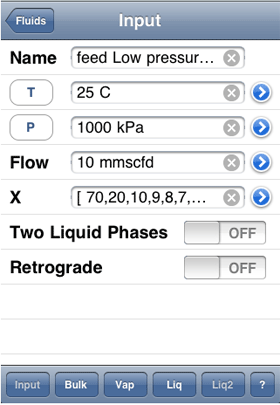Start a new case and add the compounds methane, ethane, propane, n-butane, n-pentane, n-hexane, n-heptane, n-octane. Then go to the Fluids page and add a new fluid named "feed". Essentially this is identical to the first steps of the first tutorial, except we are going to use 25 C for the temperature and 1000 kPa for the pressure. The composition will remain as

[ 70,20,10,9,8,7,6,5 ]

and the flow will again be 10 mmscfd.

Check the bulk properties.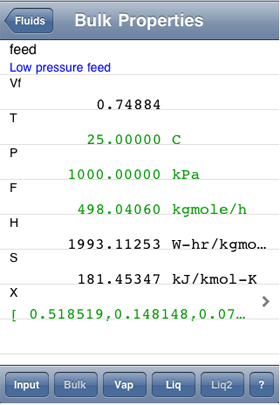They should look like those to the right.

Create another fluid.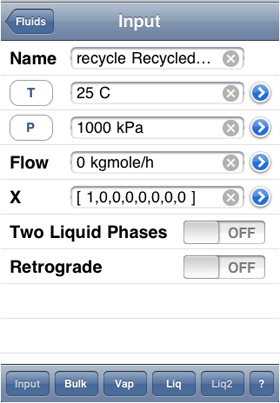Call this one "recycle" for it will represent the liquids coming back from the high pressure separator. For now give it an arbitrary temperature and pressure and a flow rate of zero. Just make the composition all methane, as the zero flow rate means it doesn't really matter as long as it has something.

Go to the diagram and create a new mixer tool.Call this "mixer" and enter the feed and recycle fluids as the feeds.

Once again create another fluid.Select P and H as the independent variables and enter #mixer into the pressure field. The rest of the fields should be filled in as in the screenshot.

Let's have a look at the bulk properties.Since the recycle flow is currently zero, these properties should be the same as for the feed fluid.

One more new fluid. It is the last one for this example, I promise.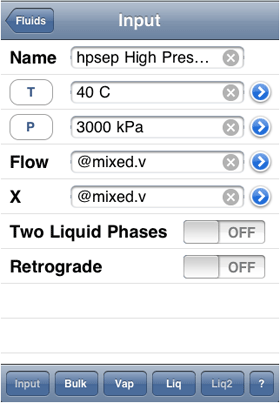We aren't really interested in the compressor or the cooler at this stage, so we can skip them and just define the HP separator fluid. Call the new fluid "hpsep" and assume a pressure of 3000 kPa and a temperature of 40 C coming out of the cooler. The flow and composition have to be the same as the vapour phase of the mixed fluid.

Once you have defined the input as in the screen shot, check the bulk properties.If all looks well, create a new Copy Values tool, using the Add Tool button on the diagram.I have named the tool "hprecycle" and set the From field to be the liquid phase of the hpsep fluid. Note that if no prefix is used, it is assumed we are copying a fluid, but it is also possible to copy a variable by prefixing the name with the dollar \$ symbol.

The To field is set to the recycle fluid.

The properties are shown for both fluids. These are the same properties selected on the Displayed Properties page, except that regardless of the settings, the mole fractions of both fluids are shown and the mass fractions are not.

Tap the Copy button to update the recycle fluid with the hpsep liquid phase values.This is a very well behaved system and already the two fluids are close in value.

Tap the Copy button again to perform another iteration.The flows are now identical, but what about those vapour fraction values. Remember that the source is the liquid phase of the hpsep fluid and for phases, the vapour fraction is interpreted as the phase fraction of that phase in the bulk fluid. The target fluid values are always the bulk values though and the vapour fraction of the recycle fluid is shown as zero, which is what you would expect given we are recycling a liquid.

Scroll down to ensure the rest of the fluid properties have converged.Apart from some very small differences in enthalpy and entropy, the fluids are pretty much identical. Try a couple of extra taps on the Copy button if you want to be really precise.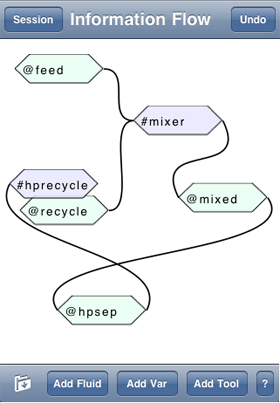For completeness, here is my little information flow diagram for this problem.

### Using the Function Solver for Recycle

Successive substitution, as employed above with the copy tool, is surprisingly effective and its simplicity makes it a good choice for straight forward problems. However when multiple recycles are involved or you are going to be making frequent changes to the problem, the manual nature of the copy tool can become cumbersome. The Function Tool introduced in the previous tutorial can be used to automate the solving of recycles, but it does require some thought as to how to frame the problem.

We will now modify our compressor recycle to use the function solver.

Add a Function Solver tool and name it solver and then bring up the formula editor for its initial function.First tap the Select Type... button and choose X as the formula type. Since this represents a composition array, this will actually add one equation to the solver for every compound in the case.

We now need to construct a formula which will represent the material errors between the previous estimate in the recycle fluid and the liquid from hpsep which is based on that estimate. These should be the compound flow rate differences, so a suitable equation might be:

(@hpsep.l*@hpsep.l.f - @recycle*@recycle.f) / @feed.f

That is the mole fraction of the hpsep liquid times its molar flow rate, minus the mole fraction of the recycle fluid times its flow rate. It is usually a good idea to normalize these things to make scale with flow rate, so I have divided by the total feed flow.The solver will have calculated the error based on the current hpsep liquid and recycle values and since we have already converged these with the Copy Tool, they will be near zero (depending on how many copies you did). However we have not yet made the recycle fluid values a function of the solver output.

Note that for formulas that return an array, the error reported is the sum of all the values in the array. Also the output value is simply reported as "Array", rather than trying to list all the values here, but you can simply create a variable referencing the solver output (#solver.0 in this case) if you wish to examine them.

Also note that we haven't taken into consideration any heat errors between the recycle fluid and the hpsep liquid. This because the hpsep fluid has a defined temperature and pressure, so we can explicitly set the recycle fluid to use those values. In the more general case where the recycled stream was having its temperature calculated, you could either supply an error based on temperature differences or on normalized heat flow differences and then use the extra output to either calculate the recycle fluid temperature or enthalpy.

Go to the recycle fluid input page.Switch the two independent variables back to T and P and enter @hpsep for both of them as we know these are constant values. Clear the formula for X and bring up its formula editor (be sure and enter something on the line before hitting the blue icon so the editor comes up rather than the mole fraction entry table).

During the solution, the solver output values may range on either side of 0, but a mole fraction cannot be negative, so our formula must be constructed to ensure only positive values result. The following formula seems to give good results:

1.5 ^ #solver.0 * @feed.l

Any value raised to the power of 0 will result in the value 1, so for the initial trial when all the output values are 0, this formula will simply result in the feed fluid liquid composition being the estimate. Increasingly negative numbers will result in mole fractions smaller than the initial estimate, but they will always remain positive. The 1.5 value is arbitrary, but provides a good balance of sensitivity. The property package will always normalize these values so they sum to 1.0.

That normalization is the reason we used compound flows as our error values in the solver rather than mole fractions and a separate error term for total flow. The normalization means that the mole fraction specification only provides n-1 degrees of freedom, where n is the number of compounds. Thus we need to be able to vary one more variable in the recycle fluid to match the n errors calculated in the solver. This will be the flow rate.

Save the composition formula and open the formula editor for the recycle flow.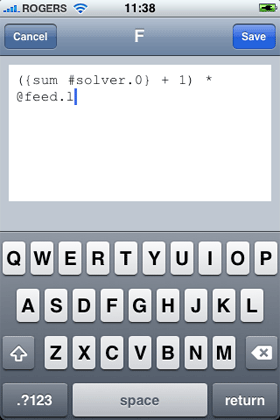Because we defined the errors in terms of compound flows, the solver will attempt to adjust outputs until those match and that means the total flows must also match. As a result of the normalization of the mole fractions done by the property package, the calculated composition is only a function of the ratios of the outputs, so the solver can adjust their combined magnitudes as appropriate to reduce the calculated errors. This means we can use an equation like the following to calculate the recycle flows from the solver output:

({sum #solver.0} + 1) * @feed.l

This sums all the output values (an array number of compounds long) and since this will initially be 0, adds one to it and multiplies the result by and estimate, in this case the feed fluid liquid phase flow. In this case we know this estimate is way too big, and just adding 0.1 might be a better match, but we will pretend we don't know that and leave it as one.which should now look like this.

Go to the bulk view to ensure the formulas are calculating correctly.The temperature and pressure should now match hpsep, while the flow and composition should be the same as the liquid from the feed fluid.The error value now appears as a non-zero value, reflecting the fact the recycle fluid no longer matches the hpsep liquid.

Turn on the Solve switch and you should see the iteration page briefly, followed by a return to the solver page with the error now reduced to 0.0002 (remember that for arrays, this is the sum of the individual errors, each of which must be below 0.0001 for convergence).

If you check the flow of the recycle fluid and that of the hpsep liquid, you will see that they are quite close in value, but the composition and enthalpy values still have significant differences. Remember that we divided the errors by the feed flow, which is about 500 kgmole/h, so each compound flow error can be as much as 500 * 0.0001 = 0.05 kgmole/h. To improve things, lets tighten the tolerance.

Open the error formula page again.We can make convergence tighter by multiplying the errors by an appropriate value. We will really tighten things up by multiplying by 1000.

(@hpsep.l*@hpsep.l.f - @recycle*@recycle.f) * 1000 / @feed.f

Save this and turn the Solve switch back on and this time the results should match very well and pretty much the results we got using the Copy Tool.

This might be a good place to mention how Alph decides what needs calculating at any given time. Essentially nothing is calculated until a value is requested. If that value depends on other properties, those will be requested and calculated if necessary. Once calculated, values are remembered until some property it depends on, directly or indirectly, is changed, at which point the remembered value is forgotten, but not recalculated until it is again requested for display or use in some other calculation. This means that these iterative calculations do not require everything to be recalculated on each iteration. Typically only those values actually required for the iteration will be calculated.

On larger calculations, you may see this effect when you switch to the Variables page. Often this page will have variables calculated from throughout the problem and so Alph will pause for a bit as it calculates everything that is needed to display the variable values. However once a variable has been calculated, it won't be recalculated unless one of the values it depends on changes.

It also means that iterative solutions should not normally perform any more calculations than are necessary on each loop.

### Using a Prebuilt Recycle Model

Some tasks, such as setting up the formulas for a recycle, will be fairly similar from problem to problem. Alph's Model Tool provides a means to encapsulate these calculations in a reusable format.

Let's modify our compressor recycle again to use a prebuilt recycle model.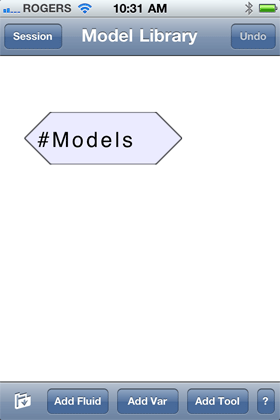The resulting Model Library case contains a single model tool, which in turn contains a number of different models. This nesting makes it easy to copy and paste the entire library into another case for easy access.

Tap the Model icon to reveal its contents. You might also want to save this case for easy access in the future.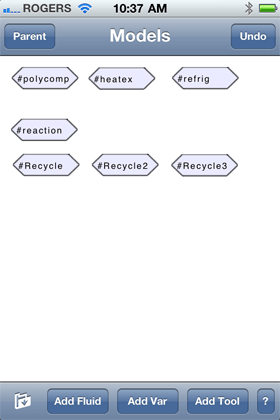Initially there are a few simple example models, but if you have calculations you use repeatedly, you can add them. Also if you have any you would like to share, please let me know.

Tap and hold on the Recycle icon and then select Copy from the resulting context menu. Reload your saved recycle cases, delete the solver tool and then tap and hold on the diagram background and then select Paste to insert the copy of the recycle model into the case.Tap on the Recycle icon to reveal its contents.A model tool is essentially a sub-flowsheet, which can contain any other fluids, tools or variables, including other models. The Next and Guess tools are Model Input tools. The names of objects in a model are completely separate from those of its parents, but the model input tools allow access to the parent's objects.

Tap on the Next icon.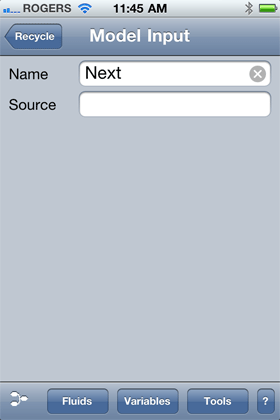The only input to this tool, other than its name, is the object to reference in the parent. Once this is filled in, then the model input tool can be referenced as if it were the referenced object.

In this case we want to reference the newly calculated estimate of the recycle fluid on each loop. This will be the liquid from the high pressure separator, namely @hpsep.l.

The similar Guess input will be the initial estimate used to start the calculation. Normally this would be a separate fluid, but in this case we can get away with just using the feed liquid - @feed.l.

Enter @hpsep.l in the Source field and then return to the recycle model, tap the Guess icon and enter @feed.l into its source field. Finally return to the recycle model and tap on the recysolve tool.An error like this should appear. It is telling us that while we removed the original solver tool, we didn't remove the references to it, specifically in the recycle fluid in the parent.

Tap the OK button and return to the recycle diagram. Note that the button at the left of the title bar will be Parent when you are in a model diagram. Tap that to return to the main diagram and then tap the recycle fluid icon.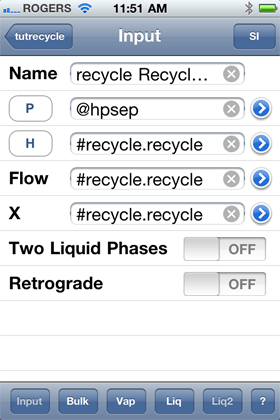Change the inputs so that P and H are the independent variables and fill in the fields as shown here.

The parent can reference any variable in a model, but not its fluids or tools directly. The recycle model has a variable named reference which has as its type Reference. A reference variable simply acts as a place holder for the object it references, in this case a fluid which also happens to be named recycle. By entering the formula #recycle.recycle, we are referencing the recycle variable of the recycle tool and when asked for a value, that variable will return the value of the fluid it references.

Normally the pressure would also be retrieved from the recycle model, but that is always just the Guess pressure and in this case we cheated and just used the feed liquid, which is at a lower pressure. For that reason we just set the pressure to be the same as the high pressure separator.

Return to the recycle model and the recysolve tool. Tap the Solve switch to start the solution.The solver should return something like this. You could return to the parent and confirm that the recycle fluid and the hpsep fluid liquid phase are essentially the same.

In most cases a better initial guess fluid will be needed to easily converge a recycle problem, especially where more than one fluid is being recycled. A useful technique is to also add a Copy Tool, and use it to update the estimate fluid(s) a couple of times before turning on the recycle solver. This not only improves the initial estimate, but gives you a better feel for whether the recycle is physically feasible.

Note that the Model Library case also has models for 2 and 3 fluid recycles and of course these could be extended or modified as needed. There are also models for basic heat exchanger balances, polytropic compressors, propane refrigeration systems and basic stoichiometric reactions.

All of these could be achieved without models, but they do provide nice shortcuts or starting points.

This brings us to the conclusion of the Alph tutorials. If you have discovered any errors of have any suggestions, please feel free to contact me.